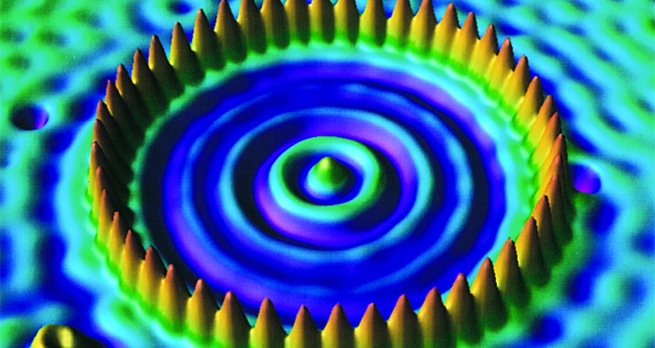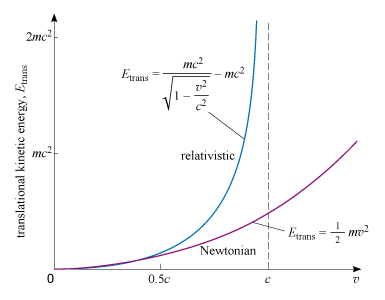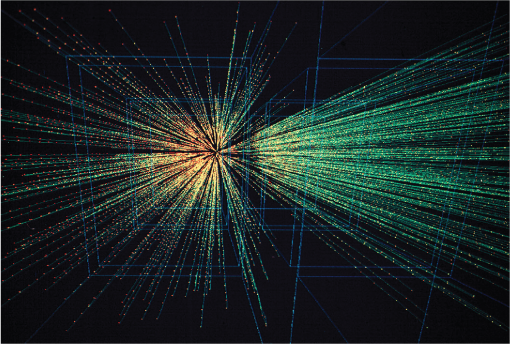Collisions and conservation laws

Start this free course now. Just create an account and sign in. Enrol and complete the course for a free statement of participation or digital badge if available.

Free course

# 7.2 Relativistic kinetic energy

One of the most celebrated aspects of special relativity is Einstein’s discovery of mass energy , the energy that a particle has by virtue of its mass. The mass energy of a particle of mass (sometimes called the rest mass in this context) is given by

Equation label: (13)

The mass energy is also known as rest energy.

The reason for mentioning this relation here is that it plays a part in determining the kinetic energy of a particle. How is this? Well, according to special relativity the total energy (including the mass energy) of a particle of mass travelling with speed is

Equation label: (14)

Since this quantity is the sum of the translational kinetic energy and the mass energy of the particle it follows that, according to the theory of relativity, the translational kinetic energy of a particle of mass and speed is

Equation label: (15)

Unlikely as it may seem, this expression actually agrees very closely with the Newtonian expression for translational kinetic energy when is small compared with . The relativistic and Newtonian definitions of translational kinetic energy are compared in Figure 7. The figure also indicates one reason why it is impossible to accelerate a particle to the speed of light; doing so would require the transfer of an unlimited amount of energy to the particle.Figure 7 The translational kinetic energy of a particle of mass plotted against the particle’s speed according to Newtonian physics and special relativity. The Newtonian relation closely approximates that of relativity for values of that are small compared with the speed of light, .

In analysing high-speed relativistic collisions , it is the relativistic expressions for momentum and energy that must be used rather than their Newtonian counterparts. In an elastic collision, all of the quantities we have just defined will be conserved:

• momentum

• mass energy

• kinetic energy

• total energy.

However, many high-energy collisions are actually inelastic, and in a high-energy inelastic collision the only quantities that are certain to be conserved are the momentum and total energy. In a general inelastic collision, neither kinetic energy nor mass energy is necessarily conserved. This means that in an inelastic collision it is quite possible for particles to be created or destroyed, thereby increasing or decreasing the mass energy. However, the conservation of total energy means that any change in mass energy must be accompanied by a compensating change in the kinetic energy. Thus particles may be created, but only at the expense of kinetic energy.

The need for kinetic energy in order to create particles explains why advances in particle physics often require the construction of powerful new particle accelerators. Increasing the kinetic energy of the colliding particles increases the mass of the particles that may be created in the collision and thus opens up the possibility of creating previously undiscovered forms of matter. Figure 8 shows the tracks of particles created in one such ‘ultra-relativistic’ collision.Figure 8 Tracks of particles coming from an ‘ultra-relativistic’ inelastic collision at CERN.

This section has made much use of the phrase ‘high-speed collision’. The meaning of the term ‘high speed’ obviously depends on context. However, if we simply take it to refer to speeds that are sufficiently high that there is a clear discrepancy between the Newtonian and relativistic values of kinetic energy and momentum, then we can say that high speed means greater than about . This may not be obvious from the curves in Figure 6 and Figure 7 because of the scale that has been used to draw them, but is the threshold used by physicists.

S217_1